# Day Calculation for Working HoursTask: Calculate the total monthly working hours for FTE's; new employees should be prorated based on their start date, employees that are leaving should be prorated based on their exit date. All absences outside the contracted allowance should be reduced from the total FTE Percentage, starting at 100%.

Dimensionalize a module by Full-Time Employees and link their Start and Exit Date from the source data. Apply time, by Day in order to determine the number of weekdays. All weekdays have a daily rate of 8 hours.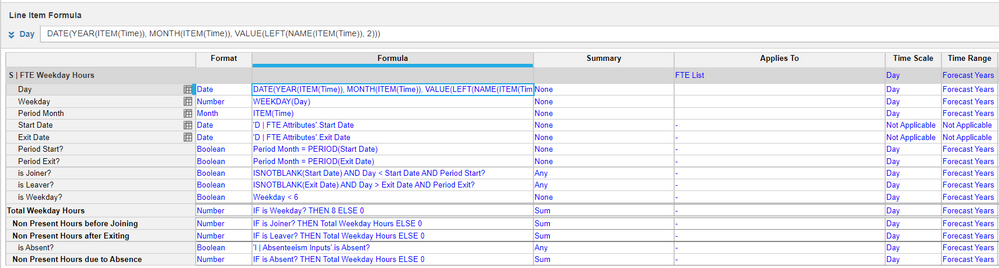Day = DATE(YEAR(ITEM(Time)), MONTH(ITEM(Time)), VALUE(LEFT(NAME(ITEM(Time)), 2)))

We use this formula because ITEM(Time) is not valid for line items applied to Day, instead, we calculate the dates for the Time Range (create time range appropriate for the forecast, usually current and N+1).

Use the Weekday Function to determine which dates are weekdays, then calculate 8 hours for all weekdays, to get the total number of working hours for each month. If Weekdays < 6 then count 8 hours.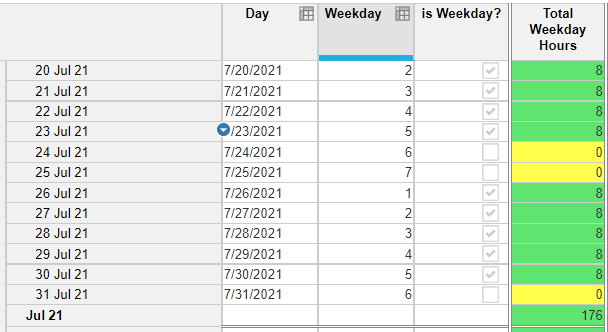Based on the logic, there are 176 working hours in the month of July.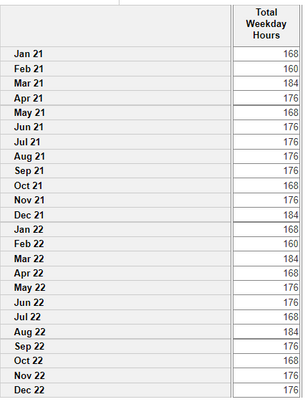As you can see from the image above, not all months have the same number of working hours.

To calculate the non-present hours for people who joined in a given month, link the start date from the source data. Count joiners if ISNOTBLANK(Start Date) AND Day < Start Date AND Period Month = PERIOD(Start Date).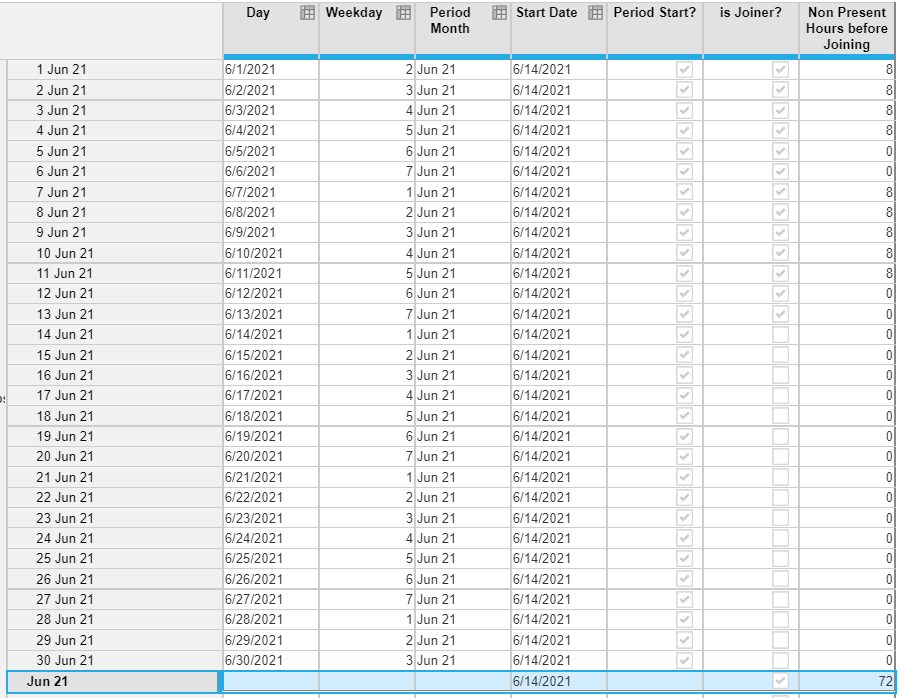In the example above, the FTE started on June 14th, therefore, the weekdays before the 14th cannot be counted in the FTE Calculation for this employee, reducing 72 hours from the total 176 hours available in June.

To calculate the non-present hours for people who left in a given month, link the exit date from the source data and count leavers if ISNOTBLANK(Exit Date) AND Day > Exit Date AND Period Month = PERIOD(Exit Date).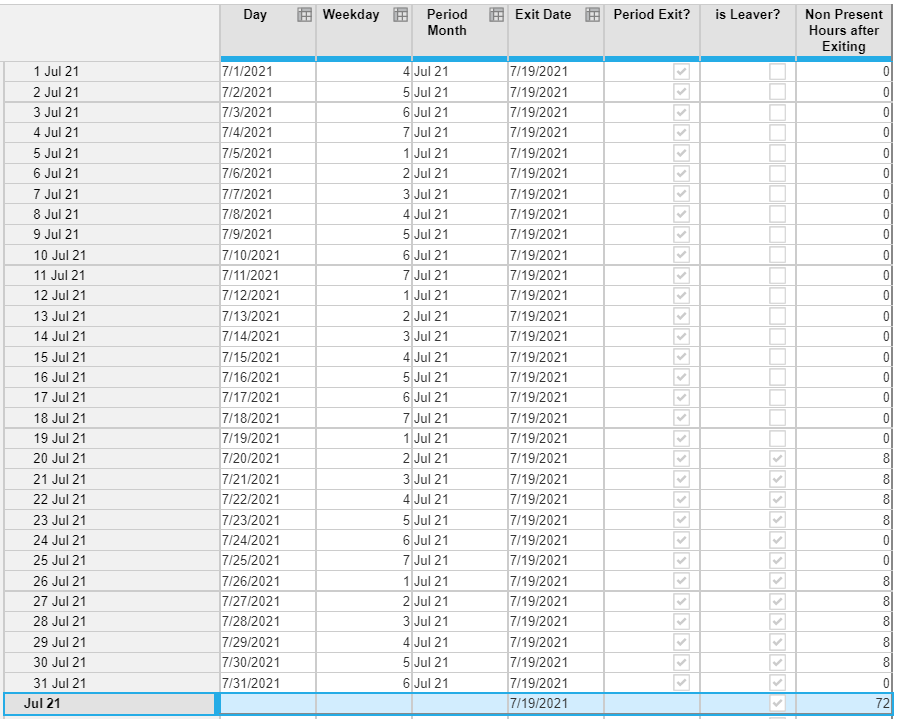In the example above, the FTE left on July 14th, therefore, the weekdays after the 19th cannot be counted in the FTE Calculation for this employee, reducing 72 hours from the total 176 hours available in July.

To calculate the number of non-present hours due to absences, create an input module to select the start and end dates of leave, or select each day using a Boolean.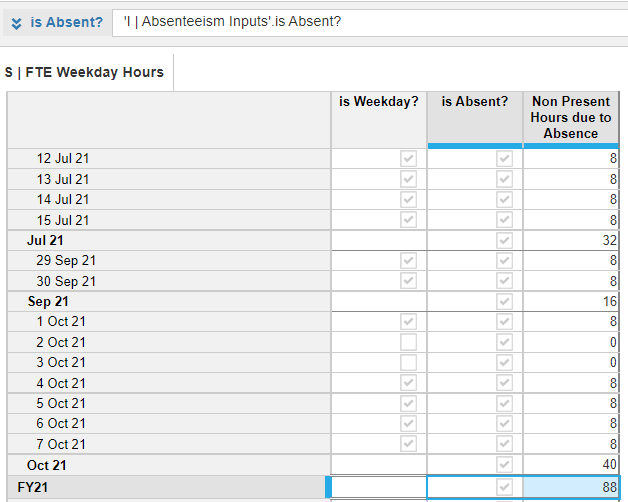The example above shows that this employee took 11 weekdays off, which means 88 hours will not be counted in the final FTE calculation.

Now that we have the total number of non-present hours for joiners, leavers, and absenteeism, we can calculate the Final FTE value for employees that joined, left, or were absent.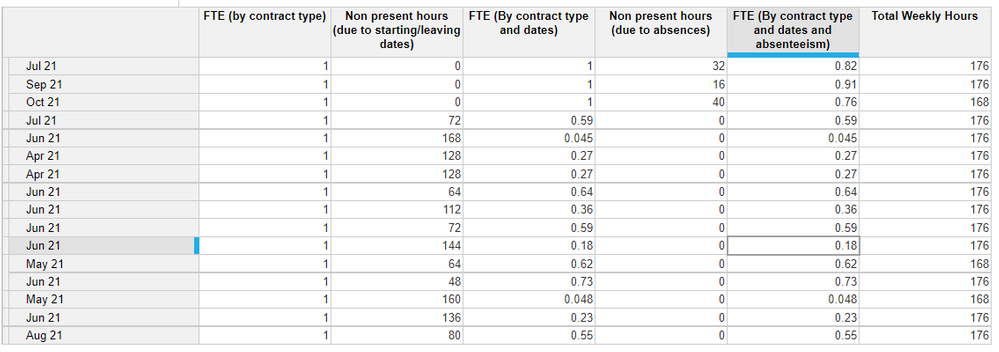All FTEs start at 100% working time and will reduce the time based on the total number of hours contracted (available) and the total number of hours worked (less non-present hours).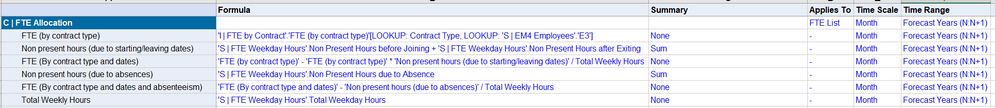The Calculation for FTEs prorated by contract dates is: 1 - (1* Non Present Hours from start/leave) / Total Working Hours (by month).

The Calculation for the final FTE value less absences is: 1 - (1* Non Present Hours from start/leave) / Total Working Hours - Non-present hours due to absences / Total Working hours (by month).

Hope this helps,

Tyler

Tagged: